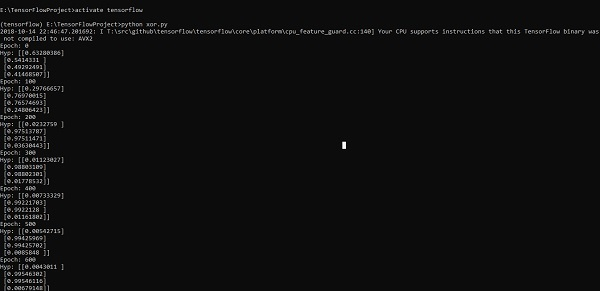# TensorFlow - XOR Implementation

In this chapter, we will learn about the XOR implementation using TensorFlow. Before starting with XOR implementation in TensorFlow, let us see the XOR table values. This will help us understand encryption and decryption process.

 A B A XOR B 0 0 0 0 1 1 1 0 1 1 1 0

XOR Cipher encryption method is basically used to encrypt data which is hard to crack with brute force method, i.e., by generating random encryption keys which match the appropriate key.

The concept of implementation with XOR Cipher is to define a XOR encryption key and then perform XOR operation of the characters in the specified string with this key, which a user tries to encrypt. Now we will focus on XOR implementation using TensorFlow, which is mentioned below −

#Declaring necessary modules
import tensorflow as tf
import numpy as np
"""
A simple numpy implementation of a XOR gate to understand the backpropagation
algorithm
"""

x = tf.placeholder(tf.float64,shape = [4,2],name = "x")
#declaring a place holder for input x
y = tf.placeholder(tf.float64,shape = [4,1],name = "y")
#declaring a place holder for desired output y

m = np.shape(x)#number of training examples
n = np.shape(x)#number of features
hidden_s = 2 #number of nodes in the hidden layer
l_r = 1#learning rate initialization

theta1 = tf.cast(tf.Variable(tf.random_normal([3,hidden_s]),name = "theta1"),tf.float64)
theta2 = tf.cast(tf.Variable(tf.random_normal([hidden_s+1,1]),name = "theta2"),tf.float64)

#conducting forward propagation
a1 = tf.concat([np.c_[np.ones(x.shape)],x],1)
#the weights of the first layer are multiplied by the input of the first layer

z1 = tf.matmul(a1,theta1)
#the input of the second layer is the output of the first layer, passed through the
activation function and column of biases is added

a2 = tf.concat([np.c_[np.ones(x.shape)],tf.sigmoid(z1)],1)
#the input of the second layer is multiplied by the weights

z3 = tf.matmul(a2,theta2)
#the output is passed through the activation function to obtain the final probability

h3 = tf.sigmoid(z3)
cost_func = -tf.reduce_sum(y*tf.log(h3)+(1-y)*tf.log(1-h3),axis = 1)

#built in tensorflow optimizer that conducts gradient descent using specified
learning rate to obtain theta values

#setting required X and Y values to perform XOR operation
X = [[0,0],[0,1],[1,0],[1,1]]
Y = [,,,]

#initializing all variables, creating a session and running a tensorflow session
init = tf.global_variables_initializer()
sess = tf.Session()
sess.run(init)

#running gradient descent for each iteration and printing the hypothesis
obtained using the updated theta values
for i in range(100000):
sess.run(optimiser, feed_dict = {x:X,y:Y})#setting place holder values using feed_dict
if i%100==0:
print("Epoch:",i)
print("Hyp:",sess.run(h3,feed_dict = {x:X,y:Y}))


The above line of code generates an output as shown in the screenshot below −